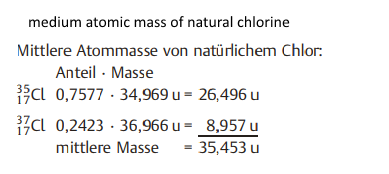# average of atomic mass unit

## Recommended Posts

In my chemistry book there is the task that I should calculate the "average of atomic mass unit" of two isotopes of chlorine. The solution is that the "atomic mass units" are calculated. Then they are added together. (Example: 26,496u + 8,957u = 35,453u). And that is already the result. But if you calculate the average, you still have to divide by two. Why I dont have to divide in this calculation?

##### Share on other sites
20 minutes ago, KlausZahn said:

In my chemistry book there is the task that I should calculate the "average of atomic mass unit" of two isotopes of chlorine. The solution is that the "atomic mass units" are calculated. Then they are added together. (Example: 26,496u + 8,957u = 35,453u). And that is already the result. But if you calculate the average, you still have to divide by two. Why I dont have to divide in this calculation?

Can you post the question?

26 and 8 are not isotpic masses for chlorine or any element.

Nor does any element have 26 protons and only 8 neutrons, or the other way round.

##### Share on other sites
34 minutes ago, studiot said:

Can you post the question?

26 and 8 are not isotpic masses for chlorine or any element.

Nor does any element have 26 protons and only 8 neutrons, or the other way round.

The question is in german. I have to calculate the medium atomic mass of natural chlorine.
The isotops are Cl-37 (24,23%) and Cl-35 (75,55%)##### Share on other sites
2 hours ago, KlausZahn said:

But if you calculate the average, you still have to divide by two. Why I dont have to divide in this calculation?

In mathematics, if you calculate average from some numbers, you indeed add them together, then divide by their quantity:  ( x1 + x2 + ... xn ) / n

But in the case of calculation of "average Chlorine mass" it's not the case, as you use percentages of abundance. They sum to one (100%). 0.7577 + 0.2423 = 1

If you would be able to count the all atoms to the single one, you could get such equation:

( 34.969u * 7577 + 36.966u * 2423 ) / 10000

(if you would have 10 thousands Chlorine atoms)

Edited by Sensei

ok, thank you

## Create an account

Register a new account